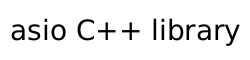### Cancellation slot requirements

A type `X` meets the `CancellationSlot` requirements if it satisfies the requirements of `CopyConstructible` (C++Std [copyconstructible]) and `Destructible` (C++Std [destructible]), as well as the additional requirements listed below.

In the table below, `x1` and `x2` denote (possibly const) values of type `X`, `mx1` denotes an xvalue of type `X`, `H` denotes a type that satisfies `CancellationHandler`, `h` denotes an xvalue of type `H`, and `u0` to `uN` denote identifiers.

Table 8. CancellationSlot requirements

expression

type

assertion/note
pre/post-conditions

`X u(x1);`

Shall not exit via an exception.

post: ```u == x1```.

`X u(mx1);`

Shall not exit via an exception.

post: `u` equals the prior value of `mx1`.

```x1 == x2```

`bool`

Returns `true` only if `x1` and `x2` can be interchanged with identical effects.
`operator==` shall be reflexive, symmetric, and transitive, and shall not exit via an exception.

```x1 != x2```

`bool`

Same as ```!(x1 == x2)```.

`x1.assign(h)`

`H&`

```x1.emplace<H>(u0, ..., uN)```

`H&`

Requires that ```is_constructible<H, decltype(u0), ..., decltype(u0)>::value``` be true.